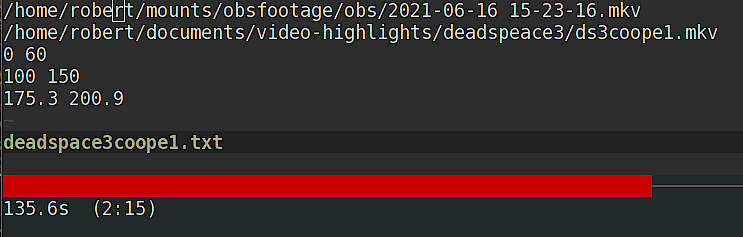# One-liner: Count total duration based on difference of begin/end`awk '! /[a-z]/ {t+=(\$2-\$1)} END {printf "%ss\t(%d:%d)\n", t, (t/60), (t%60)}' file`

Note: I write this from the perspective of an awk beginner, which I am! This is a pretty basic example, and I make some simplifications for the purposes of understanding. For further reading, see eg Awk A tutorial and Introduction, and the GNU Awk Manual.

Context: I wrote a script to go through a file with highlight times in it and create a video from that. In fact, I’ve done that several times, each with slightly different backends / purposes.

One thing I thought would be a helpful addition in making highlights is knowing the duration of highlights so far. So if I target say, 3 minutes of highlights I could quickly check how much material I’d gotten so far.

I figured I would use awk for this as I’ve seen it used for doing some relatively simple operations on text files, which is exactly what I wanted to achieve.

## Simple: Summing differences of numbers in awk

The END condition is helpful here. We can use that to get the final result of a running total. For example:

```awk { total += \$2 - \$1} END { print "output:", total }
0 60
300 600

output: 360```

will calculate the difference of the second field and the first field, and keep that in a running sum in `total`. After the end of file, we print out `total` to get the result.

Note that we aren’t outputting anything until after the end of the file, the action taken for each line is to add the difference of numbers of that line to the running total.

## Excluding Lines via Patterns

awk operates on lines, and these lines can be excluded (or included) as desired. If my highlights files were simply two columns of numbers, I could have left it as the example above. However, I start the file with the full filepath to input and output video files, which have the date and time in their filename. Those would interfere with calculations. So I would like to exclude them by specifying a pattern, which comes before the operation.

More clearly:

The essential organization of an AWK program follows the form:
pattern { action }
The pattern specifies when the action is performed.

Awk – A Tutorial and Introduction, Basic Structure

The pattern I would like is to exclude (via `!`) is any letters (via `[a-z]`). For example:

```awk '! /[a-z]/ {print \$0}'
foo
0 bar 60
300 600 baz
1000 1500
1000 1500```

I know that all my paths are lower case, or at least have a lower-case letter present, so there was no need to exclude capital letters too (`A-Z`). Regex patterns are a topic in their own right!

## Better Formatting With printf

With the two actions, above we can process a highlights file- it’ll skip lines with letters and calculate a running total of the time:

```\$ less examplehighlights.txt

/home/robert/mounts/obsfootage/obs/2021-06-16 15-23-16.mkv
0 60
100 150
175.3 200.9

\$ awk '! /[a-z]/ { total += \$2-\$1 } END { print total }' examplehighlights.txt

135.6```

That’s helpful, but the format can be improved- it would be handy to know at a glance what that is in minutes, or minutes and seconds. Here, `printf` can print a formatted string (see eg ‘How to print using awk‘).

The output I’d like is `<seconds> (<minutes>:<seconds>)`, which we can get with:

`printf "%ss\t(%d:%d)\n", total, total / 60, total % 60`

Where:

• `%s` – substitute a string here, in this case `total` seconds
• s – plain s, short for seconds
• \t – tab character
• `%d` – decimal integer (ie whole number)
• `total` – the overall sum of differences, can be a non-whole number; ie seconds
• `total / 60`, formatted as `%d` – seconds divided by 60 ie minutes, expressed as a whole number
• `total % 60`, formatted as `%d`remainder of seconds (ie modulo 60), expressed as a whole number

for example:

```\$ less examplehighlights.txt

/home/robert/mounts/obsfootage/obs/2021-06-16 15-23-16.mkv
0 60
100 150
175.3 200.9

\$awk '! /[a-z]/ { total += \$2 - \$1 } END {printf "%ss\t(%d:%d)\n", total, total / 60), total % 60}' examplehighlights.txt

135.6s  (2:15)```

## Live Count using entr

I’ve mentioned `entr` before, as a way to monitor files — for example when reconciling hledger journals (where I first came across it), or when working through videos to post on youtube — and this is a perfect place to use it!

You can work on a file (say, `examplehighlights.txt`) and have entr monitor it, running an awk command when the file is written to. I would usually have this in a split (`Ctrl+a → |` by default in byobu) below the file I am working on:

`ls examplehighlights.txt | entr -c awk '! /[a-z]/ { total += \$2 - \$1 } END {printf "%ss\t(%d:%d)\n", total, total / 60), total % 60}' /_`

here `/_` is a shortcut for the one file monitored by entr.